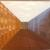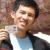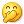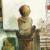## 关于c语言中scanf（）的“%c”格式控制的一个问题

phi 发布于 2013/03/18 21:02

```#include <stdio.h>
main() {
int a, b, c, temp, temp2;

a = b = c = 0;
scanf("%d", &a);
scanf("%d", &b);
temp = scanf("%d", &b);
temp2 = scanf("%c", &c);

printf("a is : %d\n", a);
printf("b is : %d\n", b);
if (c == '\n')
printf("c is  a <LF>\n");
else
printf("c is: %d(%c)\n", c, c);
printf("temp is : %d\n", temp);
printf("temp2 is : %d\n", temp2);
}```

“1 234 *“(星号('*')后面直接跟一个回车，1 和 234 还有 加号之间有个空格)时，

1 234 *
a is : 1
b is : 234
c is: 42(*)
temp is : 0
temp2 is : 1

“1 234 +”(加号后面直接跟一个回车，1 和 234 还有 加号之间有个空格)时，

a is : 1
b is : 234
c is  a <LF>
temp is : 0
temp2 is : 1

01so easy0#### 引用来自“stxy0509”的答案

0